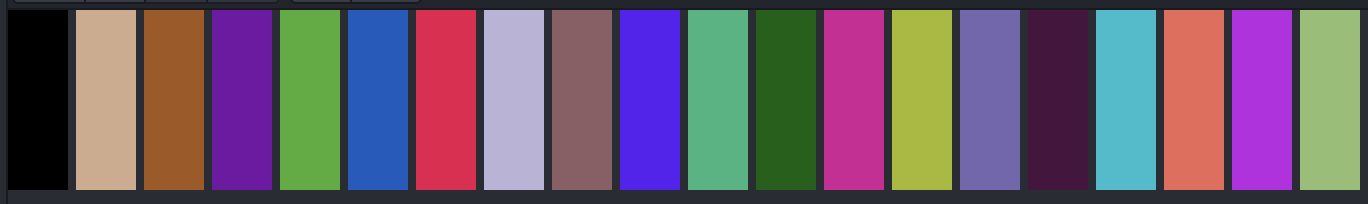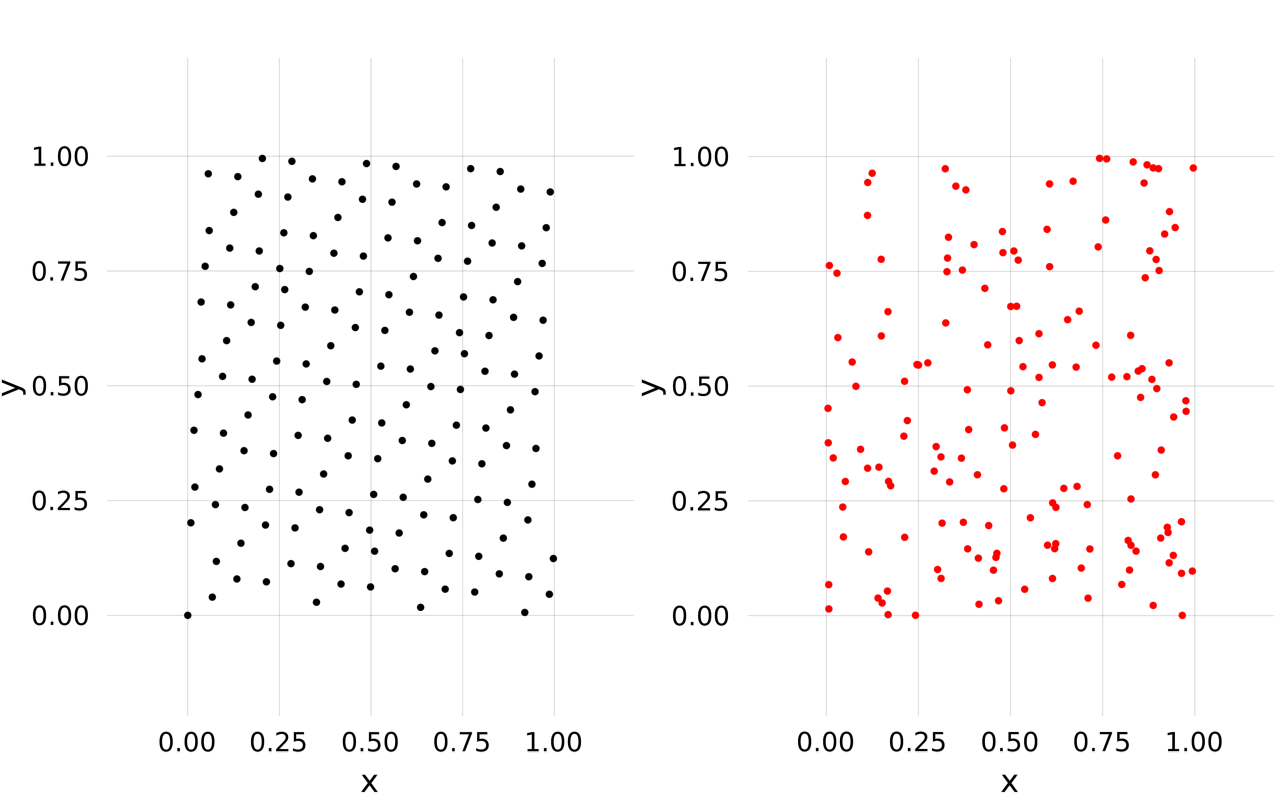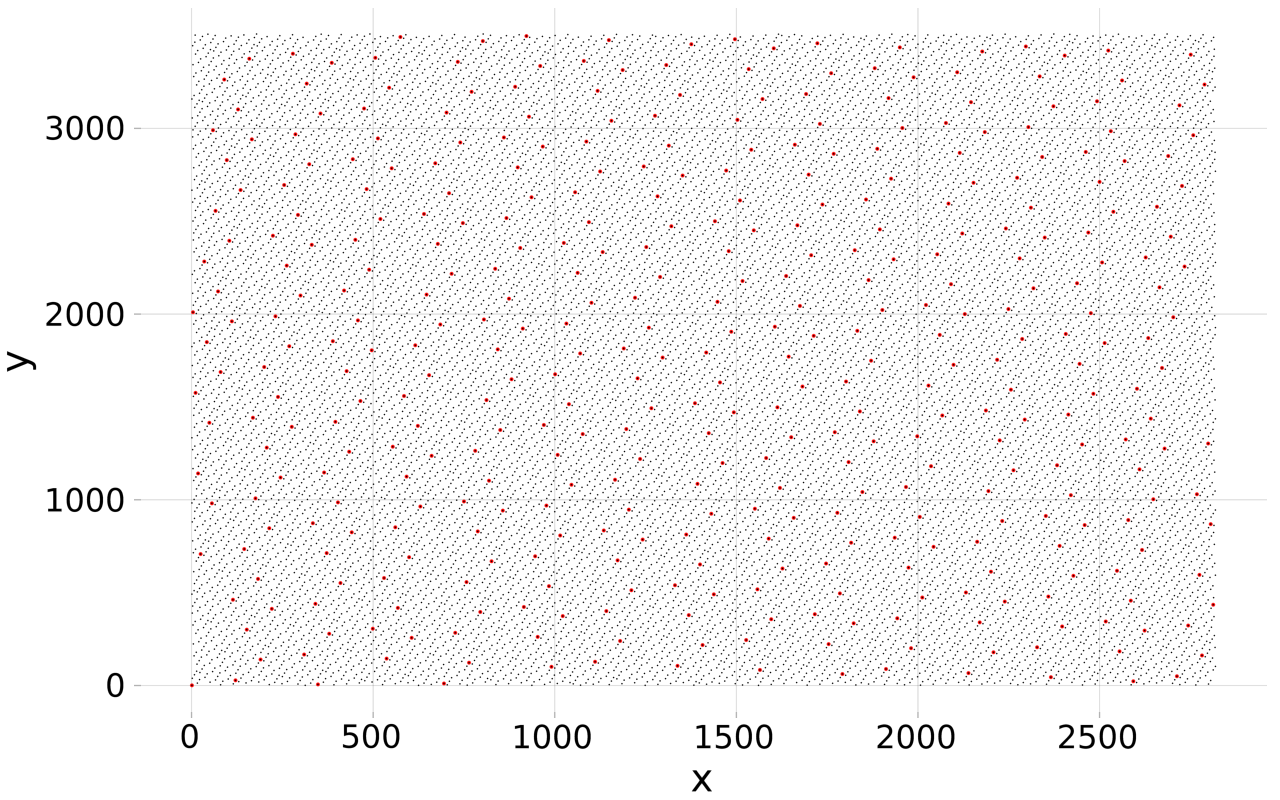## GoldenSequences.jl

Generalized golden sequences, a form of low discrepancy sequence or quasi random numbers
Author mschauer
Popularity
16 Stars
Updated Last
7 Months Ago
Started In
October 2019

# GoldenSequences.jl

Generalized golden sequences, a form of low discrepancy sequence or quasi random numbers See Martin Roberts: The Unreasonable Effectiveness of Quasirandom Sequences for background.

The `d`-dimensional sequence follows

``````x[i] = (x[i-1] .+ z) .% true, x = x0
``````

where

``````z = [ϕ[k]^(-i) for i in 1:d]
``````

and `ϕ[k]` solves `ϕ[k]^(d+1) = ϕ[k] + 1` (with `ϕ` the golden mean.)

# Golden sequence

``````julia> GoldenSequence(0.0)
0.6180339887498949
``````

Shifted golden sequence starting in 0.5

``````julia>  GoldenSequence(0.5)
0.5

julia>  GoldenSequence(0.5)
0.1180339887498949
``````

`GoldenSequence` returns an infinite iterator:

``````julia> collect(take(GoldenSequence(0.0), 10))
10-element Array{Float64,1}:
0.0
0.6180339887498949
0.2360679774997898
0.8541019662496847
0.4721359549995796
0.09016994374947451
0.7082039324993694
0.3262379212492643
0.9442719099991592
0.5623058987490541
``````

Random colors: Low discrepancy series are good choice for (quasi-) random colors

``````using Colors
n = 20
c = map(x->RGB(x...), (take(GoldenSequence(3), n))) # perfect for random colors
``````# 2D golden sequence

``````julia>  GoldenSequence(2)
(0.7548776662466927, 0.5698402909980532)
``````

As low discrepancy series these number are well distributed (left), better than random numbers (right):

```using Makie
n = 155
x = collect(Iterators.take(GoldenSequence(2), n))
p1 = scatter(x, markersize=0.02)
y = [(rand(),rand()) for i in 1:n]
p2 = scatter(y, markersize=0.02, color=:red)
vbox(p1, p2)```# Cartesian Golden Sequence

With a bit of effort, one can use Golden Sequences to generate spacefilling quasirandom sequences of cartesian indices. For example `GoldenCartesianSequence((m, m))` will create a 2D Cartesian sequence corresponding to (approximate) samples of the Golden sequence in `1:m` x `1:m`.

For that, `GoldenCartesianSequence((m, ..., m[d]))` will create full period linear congruential generators (LCG) `x[i+1] = (x[i] + c[k]) % m[k]` approximating `phi[d]^[-k]` by `c[k]/m[k]` such that `c[k]` and `m[k]` are coprime.

If `m, ..., m[d]` are coprime themselves, these `LCG` will have together period `prod(m)` and the sequence will be space filling, that is `sort(collect(take(GoldenCartesianSequence(m), prod(m)))) == CartesianIndices(m)[:]`.

This means that if `m[k]` is the denominator of a good rational approximation `c[k]//m[k] ≈ ϕ[d]^(-k)`, then the indices will be well distributed even for large `i`.

For example if `m = (2819, 3508)`:The image shows the fraction of the first 0.00005 (red) and the first 0.002 indices (black) in the `GoldenCartesianSequence((2819, 3508))`.

In short, good `m` are coprime denominators of fractions given by the function `rationalize`

```d = 2
m = @. denominator(rationalize(GoldenSequences.phis[d]^(-(1:d)), tol=0.0000001))```
``````julia> m
2-element Array{Int64,1}:
2819
3508

julia> gcd(m, m)
1
``````

There are some connections here to Knuth's multiplicative hashing method

`hash(i) = mod(i*2654435769, 2^32)`

where `2654435769` is approximately `2^32*ϕ`.

# Interface

``````GoldenSequence(n::Int) # Float64 n-dimensional golden sequence
GoldenSequence(x0::Number) # 1-d golden sequence shifted by `x0`
GoldenSequence(x0) # length(x)-d golden sequence shifted/starting in 'x0'
``````

# A flower

Flower petals grow in spots not covering older petals, the new spot is at an angle given by the golden sequence.

``````using Colors
using Makie
n = 20
c = map(x->RGB(x...), (take(GoldenSequence(3), n))) # perfect for random colors
x = collect(take(GoldenSequence(0.0), n))
petals = [(i*cos(2pi*x), i*sin(2pi*x)) for (i,x) in  enumerate(x)]
scatter(reverse(petals), color=c, markersize=10*(n:-1:1))
``````### Used By Packages

No packages found.